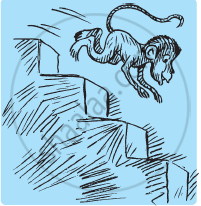Share

# A Water Tank Has Steps Inside It. a Monkey is Sitting on the Topmost Step (I.E., the First Step). the Water Level is at the Ninth Step. - CBSE Class 7 - Mathematics

ConceptIntroduction of Integer

#### Question

A water tank has steps inside it. A monkey is sitting on the topmost step (i.e., the first step). The water level is at the ninth step.1) He jumps 3 steps down and then jumps back 2 steps up. In how many jumps will he reach the water level?

2) After drinking water, he wants to go back. For this, he jumps 4 steps up and then jumps back 2 steps down in every move. In how many jumps will he reach back the top step?

3) If the number of steps moved down is represented by negative integers and the number of steps moved up by positive integers, represent his moves in part (i) and (ii) by completing the following; (a) − 3 + 2 − … = − 8 (b) 4 − 2 + … = 8. In (a) the sum (− 8) represents going down by eight steps. So, what will the sum 8 in (b) represent?

#### Solution

Let the steps moved down be represented by positive integers and the steps moved up be represented by negative integers.

1)  Initially, the monkey was at step = 1

After 1st jump, the monkey will be at step = 1 + 3 = 4

After 2nd jump, the monkey will be at step = 4 + (−2) = 2

After 3rd jump, the monkey will be at step = 2 + 3 = 5

After 4th jump, the monkey will be at step = 5 + (−2) = 3

After 5th jump, the monkey will be at step = 3 + 3 = 6

After 6th jump, the monkey will be at step = 6 + (−2) = 4

After 7th jump, the monkey will be at step = 4 + 3 = 7

After 8th jump, the monkey will be at step = 7 + (−2) = 5

After 9th jump, the monkey will be at step = 5 + 3 = 8

After 10th jump, the monkey will be at step = 8 + (−2) = 6

After 11th jump, the monkey will be at step = 6 + 3 = 9

Clearly, the monkey will be at water level (i.e., 9th step) after 11 jumps.

2) Initially, the monkey was at step = 9

After 1st jump, the monkey will be at step = 9 + (−4) = 5

After 2nd jump, the monkey will be at step = 5 + 2 = 7

After 3rd jump, the monkey will be at step = 7 + (− 4) = 3

After 4th jump, the monkey will be at step = 3 + 2 = 5

After 5th jump, the monkey will be at step = 5 + (− 4) = 1

Clearly, the monkey will reach back at the top step after 5 jumps.

3) If steps moved down are represented by negative integers and steps moved up are represented by positive integers, then his moves will be as follows.

Moves in part (i)

− 3 + 2 − 3 + 2 − 3 + 2 − 3 + 2 − 3 + 2 − 3 = −8

Moves in part (ii)

4 − 2 + 4 − 2 + 4 = 8

Moves in part (ii) represent going up 8 steps.

Is there an error in this question or solution?

#### APPEARS IN

NCERT Solution for Mathematics for Class 7 (2018 to Current)
Chapter 1: Integers
Ex. 1.10 | Q: 10 | Page no. 5
Solution A Water Tank Has Steps Inside It. a Monkey is Sitting on the Topmost Step (I.E., the First Step). the Water Level is at the Ninth Step. Concept: Introduction of Integer.
S